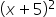Maths-
General
Easy

Question

# Explain why the middle termis 10x.Hint:

## The correct answer is: = x2 + 10x + 25

### Step 1 of 1:The given expression is: (x + 5)2Here, a=x and b=5.Here, the middle term is 10x because while expanding you have the form,In the Binomial Expansion's middle term, in the expansion of (a + b)n, there are (n + 1) terms. Therefore, we can write the middle term or terms of (a + b)n based on the value of n. It follows that there will only be one middle term if n is even and two middle terms if n is odd.
The binomial expansions of (x + y)n are used to find specific terms, such as the term independent of x or y.
Practice Questions
1. Find the expansion of (9x - 2y)12's coefficient of x5y7.
2. In the expansion of (2x - y)11, locate the 8th term.#### With Turito Foundation.#### Get an Expert Advice From Turito.